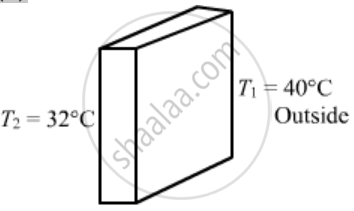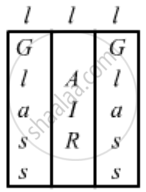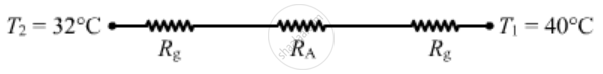Share

# A Room Has a Window Fitted with a Single 1.0 M × 2.0 M Glass of Thickness 2 Mm. (A) Calculate the Rate of Heat Flow Through the Closed Window When the Temperature Inside the Room is 32°C - Physics

ConceptHeat Transfer Conduction

#### Question

A room has a window fitted with a single 1.0 m × 2.0 m glass of thickness 2 mm. (a) Calculate the rate of heat flow through the closed window when the temperature inside the room is 32°C and the outside is 40°C. (b) The glass is now replaced by two glasspanes, each having a thickness of 1 mm and separated by a distance of 1 mm. Calculate the rate of heat flow under the same conditions of temperature. Thermal conductivity of window glass = 1.0 J s−1 m−1°C−1 and that of air = 0.025 m-1°C-1 .

#### Solution

(a)Length, l = 2 mm = 0.0002 m

Rate of flow of heat =(Delta"T")/(l/("KA"))

={1xx2xx(4032)}/{2xx10^-3}

={KA.DeltaT}/{l}

=  {1xx2xx(40-32)}/{2xx10^-3}

= 8000 J / sec

(b)|Resistance of glass, R_g = {l}/{K_g.A}

Resistance of air, R_A = {l}/{K_A.A}

From the circuit diagram, we can find that all the resistors are connected

R_s = R_g + R_A + R_g

=10^-3/2 (2/K_g + 1/K_A)

=10^-3 ( 2/1 + 1/0.025)

= 10^-3/2 xx((2xx0.025 + 1))/0.025

Rate of flow of heat ,= q ={DeltaT}/{R_5}

= ( T_1 - T_2)/R_s

= {(40-32) xx2xx0.025}/{40^_3xx(2xx0.025 + 1 )}

=381W

Is there an error in this question or solution?

#### APPEARS IN

Solution A Room Has a Window Fitted with a Single 1.0 M × 2.0 M Glass of Thickness 2 Mm. (A) Calculate the Rate of Heat Flow Through the Closed Window When the Temperature Inside the Room is 32°C Concept: Heat Transfer - Conduction.
S